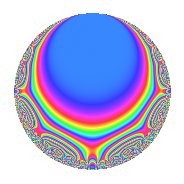# Properties

 Label 19.3.dLevel 19 Weight 3 Character orbit d Rep. character $$\chi_{19}(8,\cdot)$$ Character field $$\Q(\zeta_{6})$$ Dimension 6 Newforms 1 Sturm bound 5 Trace bound 0

# Related objects

## Defining parameters

 Level: $$N$$ = $$19$$ Weight: $$k$$ = $$3$$ Character orbit: $$[\chi]$$ = 19.d (of order $$6$$ and degree $$2$$) Character conductor: $$\operatorname{cond}(\chi)$$ = $$19$$ Character field: $$\Q(\zeta_{6})$$ Newforms: $$1$$ Sturm bound: $$5$$ Trace bound: $$0$$

## Dimensions

The following table gives the dimensions of various subspaces of $$M_{3}(19, [\chi])$$.

Total New Old
Modular forms 10 10 0
Cusp forms 6 6 0
Eisenstein series 4 4 0

## Trace form

 $$6q$$ $$\mathstrut -\mathstrut 3q^{2}$$ $$\mathstrut -\mathstrut 9q^{3}$$ $$\mathstrut +\mathstrut 5q^{4}$$ $$\mathstrut -\mathstrut 2q^{5}$$ $$\mathstrut +\mathstrut q^{6}$$ $$\mathstrut +\mathstrut 14q^{9}$$ $$\mathstrut +\mathstrut O(q^{10})$$ $$6q$$ $$\mathstrut -\mathstrut 3q^{2}$$ $$\mathstrut -\mathstrut 9q^{3}$$ $$\mathstrut +\mathstrut 5q^{4}$$ $$\mathstrut -\mathstrut 2q^{5}$$ $$\mathstrut +\mathstrut q^{6}$$ $$\mathstrut +\mathstrut 14q^{9}$$ $$\mathstrut -\mathstrut 60q^{10}$$ $$\mathstrut +\mathstrut 26q^{11}$$ $$\mathstrut +\mathstrut 30q^{13}$$ $$\mathstrut +\mathstrut 54q^{14}$$ $$\mathstrut -\mathstrut 18q^{15}$$ $$\mathstrut +\mathstrut q^{16}$$ $$\mathstrut -\mathstrut 42q^{17}$$ $$\mathstrut +\mathstrut 25q^{19}$$ $$\mathstrut +\mathstrut 108q^{20}$$ $$\mathstrut -\mathstrut 102q^{21}$$ $$\mathstrut -\mathstrut 39q^{22}$$ $$\mathstrut +\mathstrut 8q^{23}$$ $$\mathstrut -\mathstrut 83q^{24}$$ $$\mathstrut -\mathstrut 17q^{25}$$ $$\mathstrut -\mathstrut 148q^{26}$$ $$\mathstrut +\mathstrut 32q^{28}$$ $$\mathstrut -\mathstrut 12q^{29}$$ $$\mathstrut +\mathstrut 304q^{30}$$ $$\mathstrut +\mathstrut 51q^{32}$$ $$\mathstrut +\mathstrut 123q^{33}$$ $$\mathstrut -\mathstrut 6q^{34}$$ $$\mathstrut -\mathstrut 38q^{35}$$ $$\mathstrut -\mathstrut 54q^{36}$$ $$\mathstrut -\mathstrut 14q^{38}$$ $$\mathstrut -\mathstrut 44q^{39}$$ $$\mathstrut -\mathstrut 96q^{40}$$ $$\mathstrut +\mathstrut 63q^{41}$$ $$\mathstrut -\mathstrut 92q^{42}$$ $$\mathstrut -\mathstrut 34q^{43}$$ $$\mathstrut -\mathstrut 69q^{44}$$ $$\mathstrut -\mathstrut 28q^{45}$$ $$\mathstrut +\mathstrut 58q^{47}$$ $$\mathstrut -\mathstrut 147q^{48}$$ $$\mathstrut +\mathstrut 18q^{49}$$ $$\mathstrut +\mathstrut 132q^{51}$$ $$\mathstrut +\mathstrut 162q^{52}$$ $$\mathstrut -\mathstrut 12q^{53}$$ $$\mathstrut +\mathstrut 29q^{54}$$ $$\mathstrut -\mathstrut 28q^{55}$$ $$\mathstrut -\mathstrut 16q^{57}$$ $$\mathstrut +\mathstrut 172q^{58}$$ $$\mathstrut -\mathstrut 147q^{59}$$ $$\mathstrut -\mathstrut 222q^{60}$$ $$\mathstrut +\mathstrut 58q^{61}$$ $$\mathstrut -\mathstrut 116q^{62}$$ $$\mathstrut +\mathstrut 86q^{63}$$ $$\mathstrut +\mathstrut 166q^{64}$$ $$\mathstrut +\mathstrut 11q^{66}$$ $$\mathstrut +\mathstrut 201q^{67}$$ $$\mathstrut -\mathstrut 84q^{68}$$ $$\mathstrut -\mathstrut 198q^{70}$$ $$\mathstrut -\mathstrut 102q^{71}$$ $$\mathstrut +\mathstrut 210q^{72}$$ $$\mathstrut +\mathstrut 7q^{73}$$ $$\mathstrut +\mathstrut 174q^{74}$$ $$\mathstrut -\mathstrut 173q^{76}$$ $$\mathstrut -\mathstrut 376q^{77}$$ $$\mathstrut +\mathstrut 450q^{78}$$ $$\mathstrut +\mathstrut 134q^{80}$$ $$\mathstrut +\mathstrut 253q^{81}$$ $$\mathstrut -\mathstrut 145q^{82}$$ $$\mathstrut +\mathstrut 146q^{83}$$ $$\mathstrut -\mathstrut 90q^{85}$$ $$\mathstrut -\mathstrut 270q^{86}$$ $$\mathstrut -\mathstrut 568q^{87}$$ $$\mathstrut -\mathstrut 72q^{89}$$ $$\mathstrut -\mathstrut 438q^{90}$$ $$\mathstrut -\mathstrut 216q^{91}$$ $$\mathstrut +\mathstrut 72q^{92}$$ $$\mathstrut -\mathstrut 160q^{93}$$ $$\mathstrut +\mathstrut 558q^{95}$$ $$\mathstrut +\mathstrut 126q^{96}$$ $$\mathstrut +\mathstrut 21q^{97}$$ $$\mathstrut +\mathstrut 411q^{98}$$ $$\mathstrut -\mathstrut 56q^{99}$$ $$\mathstrut +\mathstrut O(q^{100})$$

## Decomposition of $$S_{3}^{\mathrm{new}}(19, [\chi])$$ into irreducible Hecke orbits

Label Dim. $$A$$ Field CM Traces $q$-expansion
$$a_2$$ $$a_3$$ $$a_5$$ $$a_7$$
19.3.d.a $$6$$ $$0.518$$ 6.0.6967728.1 None $$-3$$ $$-9$$ $$-2$$ $$0$$ $$q+(-1-\beta _{5})q^{2}+(\beta _{1}+2\beta _{2}+\beta _{3}+\beta _{5})q^{3}+\cdots$$